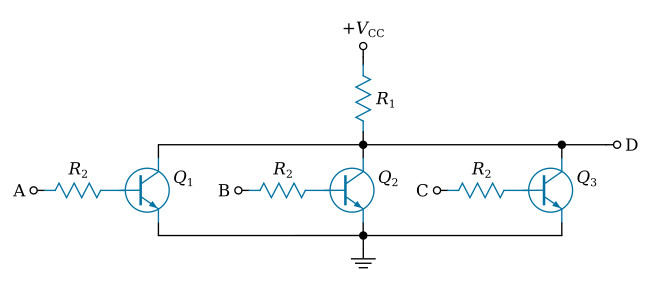Home   >   Textbooks   >   Basic Electronics   >   Logic Gates   >   Resistor-Transistor Logic (RTL)   >

Logic Gates

# Resistor-Transistor Logic (RTL)

Resistor-transistor logic (RTL) is constructed from resistors and transistors.

## One-Transistor RTL Gate

The figure below shows a simple 3-input RTL circuit which is a NOR gate. The circuit has each input combined through a coupling resistor (R1, R2, or R3) and fed to the base of Q1. The coupling resistors provide isolation that is less effective than that provided by transistors or diodes. This isolation makes the RTL sensitive to the value of the coupling resistors and the number of inputs (fan-in).Basic one-transistor RTL NOR circuit.

The operation of the circuit is quite simple. When all three inputs are zero (i.e., at ground level), the voltage VB at the base of the transistor Q1 is slightly more negative than ground since the base is tied to a negative voltage, -VBB through resistor R4. Q1 is cut off and the output voltage V0 tends to fall to the supply voltage +VCC. If any of the three inputs are positive, the current flow through its corresponding resistor pulls the voltage VB to some positive value. This drives Q1 into saturation. Here, the output voltage V0 is determined by the saturation voltage VCE(SAT) of Q1.

## Multi-Transistor RTL Gate

The multi-transistor configuration is shown in the figure below. The configuration is the same as that of the corresponding DCTL NOR circuit, except for the addition of an input resistor, R2. The addition of R2 forces some of the input voltage to appear across R2, and the base currents are more evenly divided between parallel inputs. By increasing R2, high fan-out is achieved with no current-hogging; however, as R2 is increased, the switching speed of the circuit is reduced. A design compromise must be made between high fan-out and high switching speed when using an RTL circuit.Multi-transistor RTL NOR circuit.